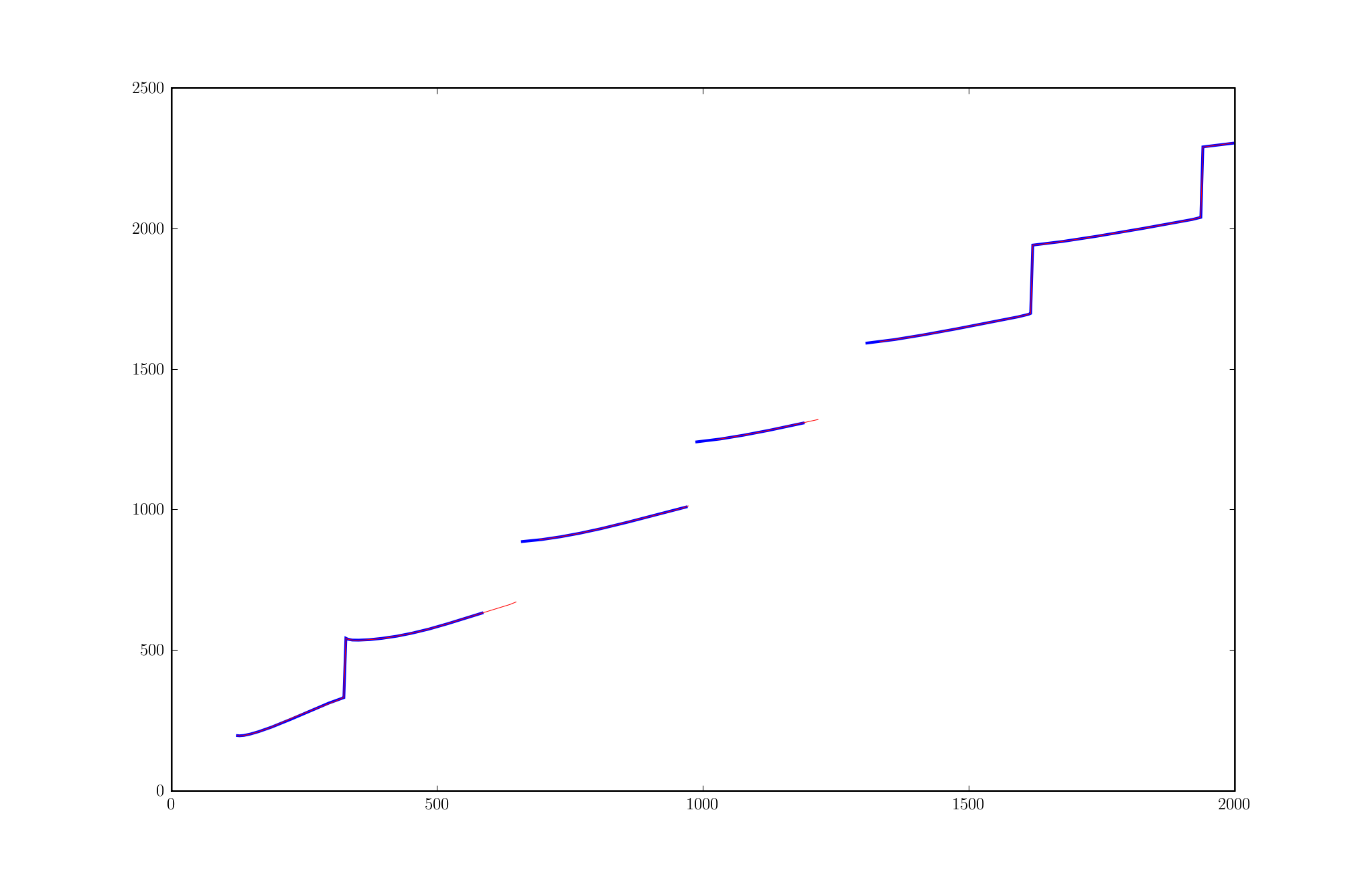# plotting data with nan

Hello,

I want to plot data containing nan values, but it seems that matplotlib
forget some non-nan values near nan values.
In the attached image, I plotted in blue the array for increasing of
x-data, and in red for decreasing x-data :

import numpy as NX

﻿In : from pylab import figure, plot, show
In : figure(2)
Out: <matplotlib.figure.Figure instance at 0x8445c8c>

In : type(Freq)
Out: <type 'numpy.ndarray'>

In : NX.any(NX.isnan(Freq))
Out: True

In : type(Grillefr)
Out: <type 'numpy.ndarray'>

In : NX.any(NX.isnan(Grillefr))
Out: False

In : plot(Grillefr, Freq, 'b', lw=2.)
Out: [<matplotlib.lines.Line2D instance at 0x9245e8c>]

In : plot(Grillefr[::-1], Freq[::-1], 'r', lw=0.5)
Out: [<matplotlib.lines.Line2D instance at 0x9245fec>]

In : show()

with Grillefr containing values from 100 to 2000.
In the matplotlibrc, I have :

Can anybody explain me why some points are not drawn by matplotlib ?···

--
Fabrice Silva <silva@...1918...>
LMA UPR CNRS 7051 - équipe S2M

Correction :

···

In the matplotlibrc, I have :

--
Fabrice Silva <silva@...1918...>
LMA UPR CNRS 7051 - équipe S2M

You didn't mention the version of matplotlib you are using. There are some known bugs using NaNs on 0.90.x and 0.91.x that have been fixed on the trunk. Unfortunately, it will be difficult to backport these fixes.

Also, can you provide a standalone example that reproduces this error (including specific data?) so that we can further investigate?

Cheers,
Mike

Fabrice Silva wrote:

···

Hello,

I want to plot data containing nan values, but it seems that matplotlib
forget some non-nan values near nan values.
In the attached image, I plotted in blue the array for increasing of
x-data, and in red for decreasing x-data :

import numpy as NX

﻿In : from pylab import figure, plot, show
In : figure(2)
Out: <matplotlib.figure.Figure instance at 0x8445c8c>
In : type(Freq)
Out: <type 'numpy.ndarray'>
In : NX.any(NX.isnan(Freq))
Out: True
In : type(Grillefr)
Out: <type 'numpy.ndarray'>
In : NX.any(NX.isnan(Grillefr))
Out: False
In : plot(Grillefr, Freq, 'b', lw=2.)
Out: [<matplotlib.lines.Line2D instance at 0x9245e8c>]
In : plot(Grillefr[::-1], Freq[::-1], 'r', lw=0.5)
Out: [<matplotlib.lines.Line2D instance at 0x9245fec>]
In : show()
with Grillefr containing values from 100 to 2000.
In the matplotlibrc, I have : maskedarray : True

Can anybody explain me why some points are not drawn by matplotlib ?

------------------------------------------------------------------------

------------------------------------------------------------------------

-------------------------------------------------------------------------
This SF.net email is sponsored by: Microsoft
Defy all challenges. Microsoft(R) Visual Studio 2008.
http://clk.atdmt.com/MRT/go/vse0120000070mrt/direct/01/
------------------------------------------------------------------------

_______________________________________________
Matplotlib-users mailing list
Matplotlib-users@lists.sourceforge.net
https://lists.sourceforge.net/lists/listinfo/matplotlib-users